Posted on

# Cantor Sect: Free Pizza & GamesPosted on

# A Marcinkiewicz-type Schur multiplier theorem in the Schatten-p class

This is combined with the Functional Analysis Seminar.
Speaker: Zhen-chuan Liu (Baylor University)
Time: Fri 11/4 3:00-3:50 PM
Location: DH 249
Title: A Marcinkiewicz-type Schur multiplier theorem in the Schatten-$p$ class.

Abstract: In this talk, we will provide a Marcinkiewicz-type condition for the (complete) boundedness of the Schur multipliers in the Schatten $p$-classes via the connection between the Fourier multipliers and Schur multipliers. As an application, we obtained an unconditional decomposition of the Schatten-$p$ class.
This talk is based on joint work with Tao Mei, Chian Yeong Chuah.
Posted on

# Generalizations of Alladi’s formula for arithmetical semigroups

Time: 10/21 Fri. 3:00-3:50 PM
Location: On zoom or at DH 249.
Speaker: Ning Ma from SUNY Buffalo
Abstract: In this talk, we will discuss a general version of Alladi’s formula with Dirichlet convolution holds for arithmetical semigroups satisfying Axiom A or Axiom A^#. As applications, we apply our main results to certain semigroups coming from algebraic number theory, arithmetical geometry and graph theory. This is a joint work with Lian Duan and Shaoyun Yi.
Posted on

# On the classification of generalized Baumslag-Solitar groups

Time: 9/23 Fri. 3:00-4:00P
Location: On zoom or at DH 249.
Speaker: Daxun Wang from SUNY Buffalo

Abstract: A generalized Baumslag-Solitar group (GBS group) is a group that acts on a tree with infinite cyclic edge and vertex stabilizers. Equivalently, it is the fundamental group of a graph of infinite cyclic groups. These groups have arisen in the study of splitting of groups. In this talk, we will discuss how to approach the group-theoretic classification of GBS groups and the current results of this problem.

Posted on

# A theorem by Peres and Schlag

Time: 9/16 Fri. 3:00-4:00PM
Location: DH 249.
Speaker: Sovanlal Mondal

Abstract: In this talk, we will prove that if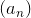is lacunary, i.e.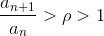for every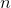, then we can find an irrational number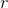such that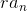(mod 1) is not dense in the one-dimensional torus. The proof that we will present in the talk was given by Yuval Peres and Wilhelm Schlag using Lovasz local lemma.
Posted on

# 2022 Faudree’s Research day

Snapshots of the 2022 Faudree’s Research dayPosted on

# 2022 Carnegie R1 Research Celebration Event

The Faculty of the Department of Mathematical Sciences has been recognized during the 2022 Carnegie R1 Research Celebration Event held by the Provost of Research Office on April 7, 2022.

Posted on

# PDE seminar

Title: Global attractors for a nonlinear boundary damped wave equation with nonlinear critical source.

Abstract: In this talk we shall consider a wave model in 3D on a bounded domain which contains nonlinear sources with critical exponent in the interior and nonlinear feedback dissipation on the boundary. Similar models with simpler nonlinear boundary terms have been already studied broadly whereas the generosity of our model is not only the presence of nonlinear damping but also nonlinear boundary source. Boundary actuators are easily accessible to external manipulations-hence feasible from the engineering point of view and practically implementable. On the other hand, the underlying mathematics is challenging. Boundary actions are represented by unbounded, unclosable operators, hence not treatable by perturbation theory(even from the point of view of well-posedness theory.)

Our main result shows that a suitably calibrated boundary damping prevents the blow up of the waves and allows to contain these asymptotically (in time) in a suitable attracting set which is compact.

Time: Apr 15, 2022 02:00 PM Central Time (US and Canada)

Join Zoom Meeting

https://memphis.zoom.us/j/89234625016?pwd=S2hGbW5CZEJKNXZVbTc4bURhR21IZz09

Meeting ID: 892 3462 5016

Passcode: 363494

Posted on

# Ergodic Theory seminar: The Lightning Model

Title: The Lightning Model

Abstract: We introduce a non-standard model for percolation on the integer lattice $\Z^2$. Randomly assign to each vertex $a \in \Z^2$ a potential, denoted $\phi_a$,  chosen independently and uniformly from the interval $[0, 1]$. For fixed $\epsilon \in [0,1]$, draw a directed edge from vertex $a$ to a nearest-neighbor vertex $b$ if $\phi_b < \phi_a + \ep$, yielding a directed subgraph of the infinite directed graph $\overrightarrow{G}$ whose vertex set is $\Z^2$, with nearest-neighbor edge set. We define notions of weak and strong percolation for our model, and observe that when $\ep = 0$ the model fails to percolate weakly, while for $\ep = 1$ it percolates strongly. We show that there is a positive $\epsilon_0$ so that for $0 \le \ep \le \ep_0$, the model fails to percolate weakly, and that when $\ep > p_\text{site}$, the critical probability for standard site percolation in $\Z^2$, the model percolates strongly. We study the number of infinite strongly connected clusters occurring in a typical configuration. We show that for these models of percolation on directed graphs, there are some subtle issues that do not arise for undirected percolation. Although our model does not have the finite energy property, we are able to show that, as in the standard model, the number of infinite strongly connected clusters is almost surely 0, 1 or $\infty$.

Location: DH 109

Time: 4/14/2022 1:00 pm.